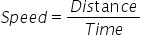Mathematics
Easy

Question

# A jet plane travels 548 miles in 4 hours. At this rate, __________ miles can be travelled in 13 hours

## 1600150015001781Hint:

## The correct answer is: 1781

### The given values of distance and time isDistance = 548 milesTime = 4 hoursTo solve the equation, we will find speed. We will find the speed by taking the ratio of distance to time. As speed is constant, we will use this to find the value of the distance travelled in 13 hours.Let’s see the formulaLet’s use the value of speed to find the second distance.We will rearrange the formula for distance. We will multiply the L.H.S and R.H.S of the equation by time.Speed × Time = DistanceDistance = Speed × Time= 137 × 13= 1781 milesTherefore, the distance travelled by the jet plane in 13 hours is 1781 miles.

The other method is to take the ratios and directly equate them. As the rate is constant, so the ratios will be constant. So we will consider the quantity we have to find as x and then equate the ratios. It will be a shortcut.

### Related Questions to study#### With Turito Foundation.#### Get an Expert Advice From Turito.